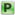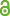Hauptmenü
• Autor
• Berkes, István
• Raseta, Marko
• TitelOn The Discrepancy And Empirical Distribution Function Of $${n_k α}$$
• Datei
• Erschienen inUniform Distribution Theory
• Band10
• Erscheinungsjahr2015
• Heft1
• Seiten1-17
• LicenceCC BY
• ISSN1336-913X
• Zugriffsrechte• AbstractBy a classical result of Philipp (1975), for any sequence $$(n_k)_{k≥1}$$ of positive integers satisfying the Hadamard gap condition, the discrepancy of $$(n_k x)_{1≤k≤N}$$ mod 1 satisfies the law of the iterated logarithm. For sequences $$(n_k)_{k≥1}$$ growing subexponentially this result becomes generally false and the asymptotic behavior of the discrepancy remains unknown. In this paper we show that for randomly sampled subsequences $$(n_k)_{k≥1}$$ the discrepancy $$D_N$$ of $$(n_k x)_{1≤k≤N}$$ mod 1 and its $$L^p$$ version $$D_N^(p)$$ not only satisfy a sharp form of the law of the iterated logarithm, but we also describe the precise asymptotic behavior of the empirical process of the sequence $$(n_k x)_{1≤k≤N}$$ , leading to substantially stronger consequences.